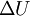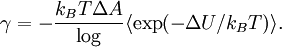# Difference between revisions of "Test area method"

The test area method is related to the test volume method for the calculation of the pressure (which, in turn, is related to Widom test-particle method). The surface tension of a planar interface is given by the change in internal energy$\Delta U$ caused by "squeezing" the system: modifying both the length in the direction normal to the interface and the area in the plane of the interface, in such a way that the total volume is left unchanged.$\gamma = - \frac{ k_B T \Delta A } \log\langle \exp(-\Delta U/k_B T)\rangle.$